Question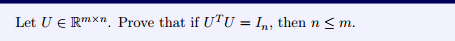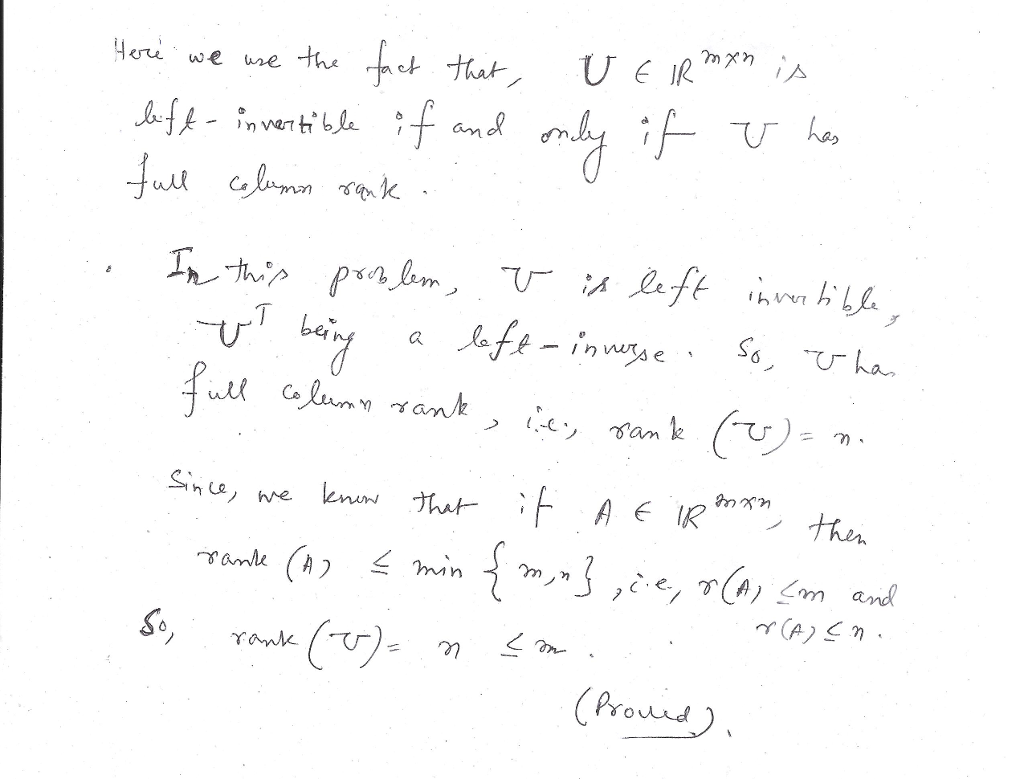#### Earn Coins

Coins can be redeemed for fabulous gifts.

Similar Homework Help Questions
• ### Let ne Nj. Prove that n < 2(6(n)).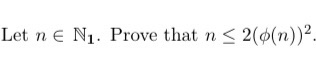Let ne Nj. Prove that n < 2(6(n)).

• ### Let A be an mx n matrix and B be an n xp matrix. (a) Prove...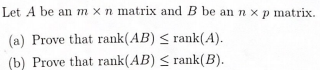Let A be an mx n matrix and B be an n xp matrix. (a) Prove that rank(AB) S rank(A). (b) Prove that rank(AB) < rank(B).

• ### let a,b > 0 . Prove that    DI < Val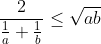let a,b > 0 . Prove that    DI < Val

• ### IDY in < oo and lim - Yn < 0o. Prove that lim,+ 1. Let In...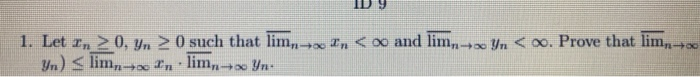IDY in < oo and lim - Yn < 0o. Prove that lim,+ 1. Let In > 0. Yn > 0 such that lim,- Yn) < lim,-- In lim,+ Yn: i tn < oo and lim yn < . Prove that lim. In 1. Let In 20, yn 0 such that lim Yn) < limn+In lim + Yr

• ### , then n lim Let Ά be a square matrix. Prove that if ρ(A)<1 Use the...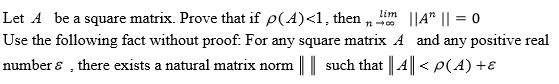, then n lim Let Ά be a square matrix. Prove that if ρ(A)<1 Use the following fact without proof. For any square matrix A and any positive real number ε , there exists a natural matrix norm I l such that l-4 ll < ρ (d) +ε IIA" 11-0

• ### 5. Prove that U(2") (n > 3) is not cyclic.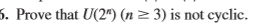5. Prove that U(2") (n > 3) is not cyclic.

• ### Let (In), and (yn).m-1 be sequences such that Pr – yn| < 1/n for all n....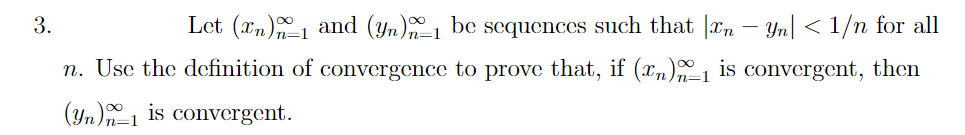Let (In), and (yn).m-1 be sequences such that Pr – yn| < 1/n for all n. Use the definition of convergence to prove that, if (2n)_1 is convergent, then (Yn)-1 is convergent.

• ### 1 4.6.3. (Harder!) Let 0 < a < 1. Prove that for any n EN, (1...1 4.6.3. (Harder!) Let 0 < a < 1. Prove that for any n EN, (1 – a)” < 1+n·a

• ### 2. (D5) Let n = o(a) and assume that a =bk. Prove that <a >=<b> if...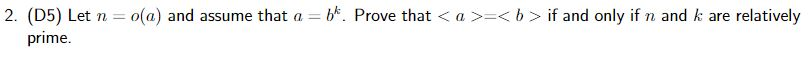2. (D5) Let n = o(a) and assume that a =bk. Prove that <a >=<b> if and only if n and k are relatively prime.

• ### real analysis. questions Prove that if lima In = 0 and > M for some M...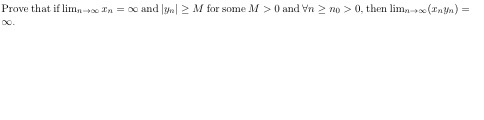real analysis. questions Prove that if lima In = 0 and > M for some M >0 and in 10 > 0, then lima (ny) - Asume 30 = 2,2-20+ In+1 = In + Prove that this sequence has a limit and find the limit. Prove that lim = L with L < if and only if every subsequence limo n L. Suppose that the sequence {an) is increasing and the sequence {yn) is decreasing. Moreover, lim a n -...